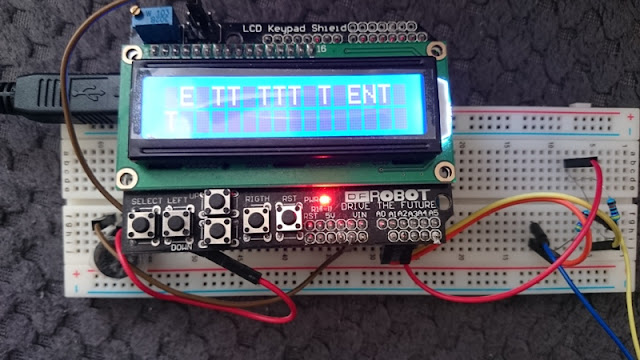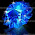## Wednesday, July 20, 2016

### See code on a character lcd  as it is being sent!Quick iambic practice keyer with arduino uno and a lcd shield

I like cw a lot and was using a straight key for most of the time. As the wrist is getting bad, started moving to automated keyers and a iambic one looks very attractive and is available in most of the transceivers. So here is a post which can be hooked up in a couple of minutes using an arduino uno (or any atmega328 board), a character lcd, a small speaker and two 470k resistors.

#### See the code on a character lcd  while sending to check errors [learners]

The idea is to use the simple and elegant touch keyer from Dimitris (SV1OBT). The keyer uses the arduino input pins to detect the touch signal. The arduino pins A1 and A2 are connected via 470k resistor to A3. The pin A1 and A2 can be connected to two small pieces of copper cladboard to form the touch key or similar to paddles. Keeping finger on one of these keys or pins produces a series of dots or dashes and moving fingers across them produce the necessary charecters and symbols. It makes it easier to key in with minimal effort. A cool video of the key can be watched on Dimitris blog (here).

I needed some way to show the letters and symbols while it is being keyed with the paddles. So i added some decoding to the keyer and added a smal lcd module to show the text. The easiest way to implemet is to use the prototyping keypad shield and an arduino uno

To generate the audio, a small speaker is connected to pin 11 (and pin 8, 9, 4, 5, 6, 7 is used by keypad shield for lcd).  The keying can be done by touching A1 or A2 pin. If both of the paddles are touched, it will alternate between dots and dashes. It can be used to key a qrp rig by adding a switching transistor /mosfet to pin13 of the arduino.

Arduino Program/Sketch

It is borrowed from dimitris code and parts of Hjalmar Skovholm Hansen OZ1JHM's excellend cw decoder.
Here is the Simple sketch. An updated version is placed at the bottom with an option to set the tone and speed (very crude version)

```/* Iambic keyer with lcd for cw practice
Courtesy SV1OBT and OZ1JHM
*/
#include <LiquidCrystal.h>
LiquidCrystal lcd(8, 9, 4, 5, 6, 7); // LCD display pins
const int colums = 16;
const int rows = 2;
int lcdindex = 0;
int line1[colums];
int line2[colums];
bool halt=false;
long startttimelow;
byte U_umlaut =  {B01010,B00000,B10001,B10001,B10001,B10001,B01110,B00000}; // 'Ü'
byte O_umlaut =  {B01010,B00000,B01110,B10001,B10001,B10001,B01110,B00000}; // 'Ö'
byte A_umlaut =  {B01010,B00000,B01110,B10001,B11111,B10001,B10001,B00000}; // 'Ä'
byte AE_capital = {B01111,B10100,B10100,B11110,B10100,B10100,B10111,B00000}; // 'Æ'
byte OE_capital = {B00001,B01110,B10011,B10101,B11001,B01110,B10000,B00000}; // 'Ø'
byte fullblock = {B11111,B11111,B11111,B11111,B11111,B11111,B11111,B11111};
byte AA_capital = {B00100,B00000,B01110,B10001,B11111,B10001,B10001,B00000}; // 'Å'
byte emtyblock = {B00000,B00000,B00000,B00000,B00000,B00000,B00000,B00000};
//#define DIT_PIN 8
//#define DAH_PIN 10
//#define EXC_PIN 9
#define DIT_PIN A1
#define DAH_PIN A2
#define EXC_PIN A3
#define LED  13
#define BAUD_DURATION  80  //mSec
#define INTERBAUD_DURATION BAUD_DURATION*1
#define INTERLETTER_DURATION  BAUD_DURATION*2 //extra time after a baud
#define DIT_DURATION BAUD_DURATION
#define DAH_DURATION BAUD_DURATION*3
#define TOUCH_THRESHOLD 5 //how long to wait in uSec, before sampling the touch pin.
#define INTERWORD_DURATION BAUD_DURATION*7
enum{
IDLE,
DIT,
DAH,
PAUSE,
};
int dit,dah;
int state;
char code;
{
digitalWrite(EXC_PIN,HIGH);
delayMicroseconds(TOUCH_THRESHOLD);
digitalWrite(EXC_PIN,LOW);
}
{
digitalWrite(EXC_PIN,HIGH);
delayMicroseconds(TOUCH_THRESHOLD);
digitalWrite(EXC_PIN,LOW);
}
void setup()
{
lcd.createChar(0, U_umlaut); // German
lcd.createChar(1, O_umlaut); // German, Swedish
lcd.createChar(2, A_umlaut); // German, Swedish
lcd.createChar(3, AE_capital); //  Danish, Norwegian
lcd.createChar(4, OE_capital); //  Danish, Norwegian
lcd.createChar(5, fullblock);
lcd.createChar(6, AA_capital); //  Danish, Norwegian, Swedish
lcd.createChar(7, emtyblock);
lcd.clear();
lcd.begin(colums, rows);
for (int index = 0; index < colums; index++){
line1[index] = 32;
line2[index] = 32;
}
Serial.begin(115200);
Serial.println("Serial is active");
pinMode(EXC_PIN,OUTPUT);
digitalWrite(EXC_PIN,LOW);
pinMode(LED,OUTPUT);
digitalWrite(LED,LOW);
state = 0;
}
void contact(unsigned char state)
{
if(state) {
digitalWrite(LED,HIGH);
analogWrite(11,127); //pin 11 drives an 8 Ohm speaker
}
else{
digitalWrite(LED,LOW);
analogWrite(11,0);
}
}
void loop()
{
switch(state){
case IDLE:
if(halt & (millis() - startttimelow) >INTERWORD_DURATION){
printascii(32);
halt=false;
startttimelow =millis();
}
if(dit) {
state = DIT;
}
else{
delayMicroseconds(30);
if(dah) {
state = DAH;
}
}
break;
case DIT:
startttimelow =millis();
contact(1);
delay(DIT_DURATION);
contact(0);
delay(INTERBAUD_DURATION);
Serial.print(".");
strcat(code,".");
//now, if dah is pressed go there, else check for dit
if(dah){
state = DAH;
}
else{
if(dit) {
state = DIT;
}
else {
delay(INTERLETTER_DURATION);
Serial.print("|");
docode();
code = '\0';
state = IDLE;
}
}
break;
case DAH:
startttimelow =millis();
contact(1);
delay(DAH_DURATION);
contact(0);
delay(INTERBAUD_DURATION);
Serial.print("-");
strcat(code,"-");
if(dit){
state = DIT;
}
else{
if(dah) {
state = DAH;
}
else {
delay(INTERLETTER_DURATION);
Serial.print("|");
docode();
code = '\0';
state = IDLE;
}
}
break;
}//switch
delay(1);
}
void docode(){
if (strcmp(code,".-") == 0) printascii(65);
if (strcmp(code,"-...") == 0) printascii(66);
if (strcmp(code,"-.-.") == 0) printascii(67);
if (strcmp(code,"-..") == 0) printascii(68);
if (strcmp(code,".") == 0) printascii(69);
if (strcmp(code,"..-.") == 0) printascii(70);
if (strcmp(code,"--.") == 0) printascii(71);
if (strcmp(code,"....") == 0) printascii(72);
if (strcmp(code,"..") == 0) printascii(73);
if (strcmp(code,".---") == 0) printascii(74);
if (strcmp(code,"-.-") == 0) printascii(75);
if (strcmp(code,".-..") == 0) printascii(76);
if (strcmp(code,"--") == 0) printascii(77);
if (strcmp(code,"-.") == 0) printascii(78);
if (strcmp(code,"---") == 0) printascii(79);
if (strcmp(code,".--.") == 0) printascii(80);
if (strcmp(code,"--.-") == 0) printascii(81);
if (strcmp(code,".-.") == 0) printascii(82);
if (strcmp(code,"...") == 0) printascii(83);
if (strcmp(code,"-") == 0) printascii(84);
if (strcmp(code,"..-") == 0) printascii(85);
if (strcmp(code,"...-") == 0) printascii(86);
if (strcmp(code,".--") == 0) printascii(87);
if (strcmp(code,"-..-") == 0) printascii(88);
if (strcmp(code,"-.--") == 0) printascii(89);
if (strcmp(code,"--..") == 0) printascii(90);
if (strcmp(code,".----") == 0) printascii(49);
if (strcmp(code,"..---") == 0) printascii(50);
if (strcmp(code,"...--") == 0) printascii(51);
if (strcmp(code,"....-") == 0) printascii(52);
if (strcmp(code,".....") == 0) printascii(53);
if (strcmp(code,"-....") == 0) printascii(54);
if (strcmp(code,"--...") == 0) printascii(55);
if (strcmp(code,"---..") == 0) printascii(56);
if (strcmp(code,"----.") == 0) printascii(57);
if (strcmp(code,"-----") == 0) printascii(48);
if (strcmp(code,"..--..") == 0) printascii(63);
if (strcmp(code,".-.-.-") == 0) printascii(46);
if (strcmp(code,"--..--") == 0) printascii(44);
if (strcmp(code,"-.-.--") == 0) printascii(33);
if (strcmp(code,".--.-.") == 0) printascii(64);
if (strcmp(code,"---...") == 0) printascii(58);
if (strcmp(code,"-....-") == 0) printascii(45);
if (strcmp(code,"-..-.") == 0) printascii(47);
if (strcmp(code,"-.--.") == 0) printascii(40);
if (strcmp(code,"-.--.-") == 0) printascii(41);
if (strcmp(code,".-...") == 0) printascii(95);
if (strcmp(code,"...-..-") == 0) printascii(36);
if (strcmp(code,"...-.-") == 0) printascii(62);
if (strcmp(code,".-.-.") == 0) printascii(60);
if (strcmp(code,"...-.") == 0) printascii(126);
if (strcmp(code,".-.-") == 0) printascii(3);
if (strcmp(code,"---.") == 0) printascii(4);
if (strcmp(code,".--.-") == 0) printascii(6);
halt=true;
}
void sprintascii(int asciinumber){
Serial.println(char(asciinumber));
}
void printascii(int asciinumber){
sprintascii(asciinumber);
int fail = 0;
if (rows == 4 and colums == 16)fail = -4;
if (lcdindex > colums-1){
lcdindex = 0;
if (rows==4){
for (int i = 0; i <= colums-1 ; i++){
lcd.setCursor(i,rows-3);
lcd.write(line2[i]);
line2[i]=line1[i];
}
}
for (int i = 0; i <= colums-1 ; i++){
lcd.setCursor(i+fail,rows-2);
lcd.write(line1[i]);
lcd.setCursor(i+fail,rows-1);
lcd.write(32);
}
}
line1[lcdindex]=asciinumber;
lcd.setCursor(lcdindex+fail,rows-1);
lcd.write(asciinumber);
lcdindex += 1;
}
```

### Updated Version of iambic keyer-decoder with tone and speed selection

Here is slightly modified version with an option to set the speeds based on the keypad shield buttons. Very crude and quick version.  Edit the tone value for a pleasant sounding audio from the speaker.

1.hi, i have test this project and at first it worked but after 30 min the iambric keyer is failing because every singular touch in a . or - interpreting this like T or E doesnt takes the S neither the O
what happens? maybe is the 470k resistors?

1.Perhaps give a try with touching the ground using the other hand and see if it helps.

2.solved! thank you very much!

2.do you know how to modify the code in order to make more time betwen . and - for introduce a character its because i need some second for introduce a leter like c and my key wont be "tactile"

1.Change the value of #define BAUD_DURATION 80 to adjust the speed. Smaller values like 50 or 40 means faster sending. You can increase it to 100 or 120 to slow down.

2.Concentrate on the sounds rather than buttons and gradually you will get adjusted to send it faster. Good luck

3.sorry the time whic i want to increase is the one between a . and a -

3.ok i have modified it better slower...
another thing that i would like to change is the sound of the speaker im using a 4ohm and 5w and i would like it to sound with some eco like the real one for example the sound of this videohttps://www.youtube.com/watch?v=XqYSTWDYDA4 it is possible?

and do you have some modification in order to introduce the characters (letters) into the lcd with the buttoms of the shield in order to encode a text and send it with the speaker and led by morse?
thanks again!

1.Good question :)

See modified one below. Adjust #define TONE 400 to 500 or 600 etc to get a nicer sound for your ears.

73s

Speed can be adjusted with the key pad. Very crude one but it works

2.there isnt a #define TONE in the code, i have to create it?
and how can i adjust it?
what i want to do with the keypad is to introduce a text in order to transmite it by morse like this link:https://www.youtube.com/watch?v=0qfc1B6O2EA&t=2s

3.See the updated code in the blog post(scroll down to the bottom, it is placed after the old code).

or see: https://gist.githubusercontent.com/riyas-org/f8203c4f3afe42acf51f02c45523f055/raw/349ea67307b7ae30baac6170d8739a805ee7ea88/iambickeyer_tone_speed.ino

4.sorry i havent seen the second version of the code, i was able to modify this thanks
another question, there is a way to introduce a pre encoded message into the code and reproduce it by led and speaker by morse with a buttom it is for example to say hello in morse jus press a buttom connected into the digital pin 12, to say how are you press the bottom connected into digital pin 10 etc?
how can i do it for send morse messages quickly?

1.Currently not implemented. But my original plan was to add this keyer together with features from https://blog.radioartisan.com/arduino-cw-keyer/
Have it in my wish list. But later when i get more free time.
Regards

5.Ok i wish yo do that because is a very nice project
I have used you second version of the code but in the lcd doesnt appears any character when i touch the keyer

6.Hello i tested your code, it works ok, but some problems:
1. in your enum, you use PAUSE. That is not used anywhere?
2. I have a 20x4 display and chanke the rows and cols respectivly. The lines jump upwards but the first row is always empty, so there are only 3 rows in use. What is the problem here?